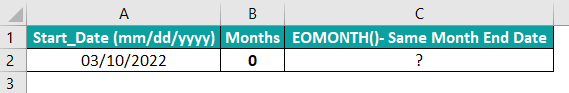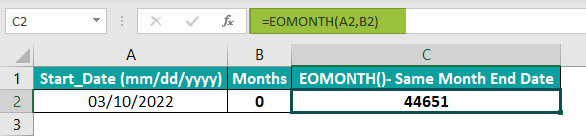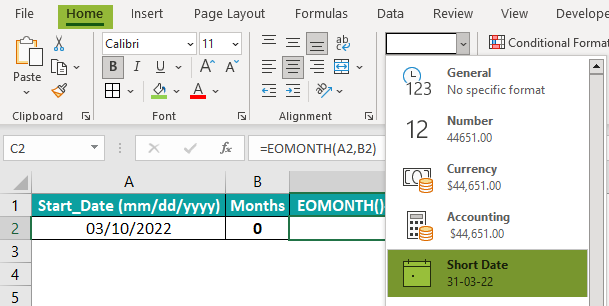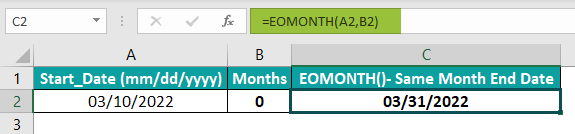# EOMONTH In ExcelArticle byExcelMojo Team## What Is EOMONTH Function In Excel?

The EOMONTH function in Excel is an inbuilt Date & Time function which returns the serial number of the last day of the month based on the supplied start date. Users can use the function EOMONTH in Excel while calculating the maturity or due dates falling on the last day of the specific month.

For example, the below table contains a list of dates entered using the DATE(), and the comments show the dates we need to calculate using EOMONTH in Excel.

Apply the EOMONTH in Excel formula in the column D cells and update their Number Format in the Home tab as Short Date to get the below output.

In the above table, column D shows the last day of the month, based on each specified start date and corresponding months. And the EOMONTH() will return the serial number of the month’s end date. But once we select the target cells and set their Number Format in the Home tab as Short Date, we will see the resulting dates in the proper format.

Thus, in this way, using EOMONTH in Excel, we can determine the month’s end date from the specified start date.

###### Key Takeaways
• The function EOMONTH in Excel returns the serial number for the month’s last day, based on the provided start date. Thus, we can use the function to determine maturity or due dates falling on the month’s last day.
• The EOMONTH() takes two mandatory arguments as inputs, start_date and months.
• Typically, we should enter the start_date argument value using functions such as DATE() to ensure the start_date has the proper Date format.
• We can apply the EOMONTH() to determine the first day of a month or the number of days in a month. Also, using EOMONTH() with other Excel functions such as IF, AND, and SUMIFS yields impressive results.

### EOMONTH() Excel Formula

The EOMONTH formula in excel is:

where,

• start_date: The starting date in the Date which we must enter the dates using date functions, such as DATE.
• months: The number of months earlier or after the starting date. This argument will be a positive value to get a future date and a negative value to get a past date.

Both the arguments mentioned in the EOMONTH in Excel syntax are mandatory.

Please Note: While applying the EOMONTH Formula in Excel, we might not get the desired results if the start_date argument is not a date. On the other hand, if the argument months is not an integer, the EOMONTH() will return the #VALUE! error. Also, if start_date is an invalid date or the resulting date from the addition of start_date and months is invalid, the function EOMONTH in Excel will return the #NUM! error.

### How To Use EOMONTH Excel Function?

The steps to use the function EOMONTH in Excel are:

1. First, ensure the start dates in the source data have the Number Format set as Date.

2. Select the target cell, enter the EOMONTH in Excel formula, and press Enter.

3. Select the target cell containing the serial number for the determined date and set the Number Format as Date to achieve the last day of the month in the proper date format.

Let us understand the above steps to use EOMONTH in Excel with example.

Consider the below table. It contains a set of dates, in the Date format, and the months we need to add to the dates to get the required EOMONTH in Excel result in each cell.

The steps are:

Step 1: Select cell C2, enter the EOMONTH() formula mentioned in the Formula Bar in the below image, and press Enter.

Alternatively, we can select cell C2, then click Formulas > Date & Time > EOMONTH to open the Function Arguments window.

Update the arguments in the Function Arguments window.

And once we click OK, we will get the EOMONTH() return value in cell C2.

Step 2: Choose cell C2 and set the Number Format in the Home tab as Short Date.

Once we select Short Date, the serial number for the date gets converted into the proper date format.

Step 3: Drag the fill handle downwards to copy the formula in cells C3:C7.

Every Months value gets added to the corresponding initial or start date. For example, in row 3, 5 months get added to the second month in the initial date, and thus the last day of July or the seventh month becomes the EOMONTH in Excel result in cell C3. Likewise, in row 5, one month gets deducted from 4, or April, in the initial date, thus making the last day of March the EOMONTH() output in cell C5.

### Examples

Now that we have seen the steps to use the function EOMONTH in Excel with example, here are a few ways to use it to achieve the desired results.

#### Example 1

Suppose we want to determine the first day of a month, given the current date. Then, we can apply the EOMONTH with TODAY Excel Function to get the required data.

Consider the below table. It contains the requirements to find out the first day of a month from the current date.

The steps to use the functions EOMONTH in Excel and TODAY are.

Step 1: Select cell B2, enter the formula provided in the Formula Bar in the below image, and press Enter.

Step 2: Select cell B2 and set the Number Format in the Home tab as Short Date.

Once we select Short Date, the serial number for the date gets converted into the proper date format.

Step 3: Enter the required formulas in cells B3:B6 as explained in steps 1 and 2. The formulas we need to apply to fulfill each requirement are as mentioned in column C.

In this example, the current date is 21-09-22, which the TODAY() will return. So the EOMONTH() determines the month’s last day by adding or deducting months to or from the current date. And the term one adds a day to the EOMONTH() return value to give the first day of the required month from the current date.

#### Example 2

We can determine the number of days in the month based on the given date. The EOMONTH with d can help us create a simple formula to get the required data.

Suppose we have a table with a list of dates, entered using the DATE Excel function, as depicted in the below image. And the dates are in mm/dd/yyyy date format.

The steps to find out the total number of days in the month, using the functions EOMONTH in Excel with DAY(), are:

Step 1: Select cell B2, enter the formula provided in the Formula Bar in the below image, and press Enter.

Step 2: Drag the fill handle downwards to copy the formula in cells B3:B8.

First, the EOMONTH() returns the last day of the specific month in which the given date lies. Then, the DAY() returns the day of the determined date as an integer. For example, cell A2 contains an October month date. So the EOMONTH() returns the value 10/31/2022, the last day in October. And then, the DAY() returns the day of the date, 31, as the output in cell B2.

#### Example 3

In this example, we shall see how we can use EOMONTH with IF and AND Excel function.

Consider the below table.

Suppose cell B1 contains the current date, and column C contains bill-generated dates. And our goal is to determine billing amounts pending for less than or equal to 15 days, 16-45 days, and equal to or more than 46 days in the cell range F4:H10.

Using the current and bill-generated dates, we can apply the functions EOMONTH in Excel, IF, and AND to populate the required data in the target cells, F4:H10. And the steps are:

Step 1: Select cell F4, enter the below formula, and press Enter.

=IF(EOMONTH(\$B\$1,0)-C4<=15,B4,””)

Step 2: Drag the fill handle downwards to copy the formula in cell range F5:F10.

Step 3: Select cell G4, enter the below formula, and press Enter.

=IF(AND(EOMONTH(\$B\$1,0)-C4>=16,EOMONTH(\$B\$1,0)-C4<=45),B4,””)

Step 4: Drag the fill handle downwards to copy the formula in the range G5:G10.

Step 5: Select cell H4, enter the below formula, and press Enter.

=IF(EOMONTH(\$B\$1,0)-C4>=46,B4,””)

Step 6: Drag the fill handle downwards to copy the formula in cells H5:H10.

Step 7: Select the cell range F4:H10 and set the Number Format in the Home tab as Currency.

Once we click on Currency, the pending billing amounts will be in the proper currency format.

The EOMONTH() returns the last day of the current month based on the current date in cell B1. The IF() in each target cell checks under which category, mentioned in cells F3:H3, the difference between the current month’s last day and the bill-generated date fall. Accordingly, the pending billing amounts get populated in the respective cells in the cell range F4:H10.

### Important Things To Note

• We need to convert the EOMONTH() output, the serial number of the last day of the month, to proper data format using the Number Format settings in Home tab.
• If the argument months in the function EOMONTH in Excel is a decimal value, the function adds only the integer part to start_date.
• Suppose the start_date is invalid or the calculated last day of the month by adding or deducting the specified number of months to or from the given start date is not valid. Then, the EOMONTH() returns #NUM! error.
• If the start_date or months is non-numeric, the EOMONTH() will return #VALUE! error.

What value will be used in the months section in the EOMONTH formula to get the same month end date?

The value used in the months section in the EOMONTH formula to get the same month end date will be 0.

Suppose we need to get the same month’s end date for the start date mentioned in the below table.The steps are:

Step 1: Select cell C2, enter the EOMONTH() provided in the Formula Bar in the below image, and press Enter.Step 2: Select cell C2 and set the Number Format in the Home tab as Short Date.Once we click on Short Date, the EOMONTH() return value in cell C2 will be in the proper date format.The month in the start date is 3 or March. And as the argument months supplied to the EOMONTH() is 0 (cell B2), its last day, 3/31/2022, gets displayed in the target cell C2.

Why is my EOMONTH formula not working?

The EOMONTH formula may not work due to the below reasons:

1. The specified start_date, or resulting date, obtained by adding or deducting the number of months to or from the start_date is invalid.
2. The supplied arguments to the function are non-numeric.

When to use EOMONTH in Excel?

We can use EOMONTH in Excel when we have to:

1. Determine maturity dates or due dates falling on the month’s end date.
2. Calculate the number of days in a month based on the given start_date.
3. Determine the first day of a specific month from the start_date.